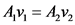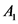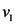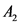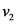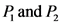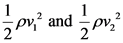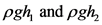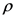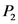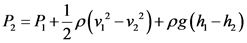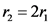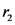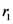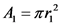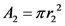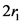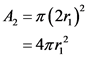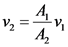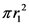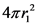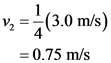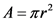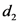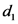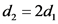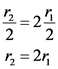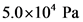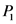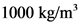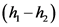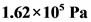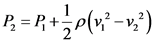# At one point in a pipeline the water’s speed is 3.00m/s and the gauge pressure is 5.00×104pa.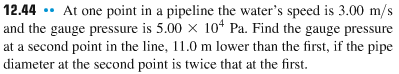Show All Work For Credit At one point in a pipeline the water’s speed is 3.00 m/s and the gauge pressure is 5.00 times 104 Pa. Find the gauge pressure at a second point in the line, 11.0 m lower than the first, if the pipe diameter at the second point is twice that at the first.

General guidance

Concepts and reason
The concepts used to solve this problem are Bernoulli’s theorem and equation of continuity.
First, calculate the speed of the water at second point in the line by using the continuity equation. Finally, calculate the gauge pressure at a second point in the line by using the Bernoulli’s equation.

Fundamentals

The equation of continuity states that when the fluid is in motion, then the motion of the fluid must be in such a way that mass is conserved. The equation of rate of flow of liquid is given as follows:

Here, is the area of cross section at point 1, is the speed of liquid at point 1, is the area of cross at point 2 and is the speed of liquid at point 2.
The Bernoulli equation is the conservation of energy principle for the flowing fluids. The equation of the Bernoulli principle is given as follows:

Here, is the pressure at point 1 and 2, is the kinetic energy per unit volume at point 1 and 2, are the potential energies at point 1 and 2, is the density, g is the acceleration due to gravity, and h is the height.
Rearrange the above equation for.

Step-by-step

Step 1 of 2

The diameter of the pipe at the second point is twice that at the first. So, the radius of the pipe at the second point is also twice that at the first.

Here, is the radius of the pipe at the second point and is the radius of the pipe at the first point.
The cross-sectional area of the pipe at first point is,

The cross-sectional area of the pipe at second point is,

Substitute for.

The equation of continuity is given as follows:

Rearrange the above equation for.

Substitute forand for.

Substitute 3.00 m/s for in the above equation.

The equation of continuity states that the product of area of cross section and speed of the liquid is same at both the points.
The area of the pipeline is,

Here, r is the radius of the pipeline.
Let be the diameter of the pipe at a second point and be the diameter of the pipe at a first point. Given that, the diameter at second point is twice that at the first point. The diameter at the second point is,

The radius of the pipe at any point is equal to one half of its diameter. So, the radius at the second point is,

Do not consider the equation of continuity. It is incorrect because the correct formula is.

Use the Bernoulli’s equation to calculate the gauge pressure at a second point in the line.

Step 2 of 2

The expression for the gauge pressure at a second point in the line is,

Substitute for , for , 3.0 m/s for , 0.75 m/s for and 11.0 m for in the above equation.

The gauge pressure at a second point in the line is.

The height difference between both the points is given as 11.0 m. the Bernoulli Effect is the lowering of fluid pressure in regions where the flow velocity is increased. Bernoulli’s theorem states that the total initial energy per unit volume of the fluid is equal to the total final energy per unit volume of the fluid.

Do not use the formulato obtain the gauge pressure at a second point in the line. It is incorrect because the correct formula for the gauge pressure at a second point in the line is.

The gauge pressure at a second point in the line is.

The gauge pressure at a second point in the line is.

Av = 4,2
480 + \$0 \$+ ‘=’y8d +,n0 +
P, and P,
pgh and pgh,
(14=’4)3d+(439–), 4+4=
1₂ = 2r,
Α = πη
Α, = πη?
A2 = 1 (21) = 49r;
Av = 4,2
ニーリ
v2 = +(3.0 m/s) = 0.75 m/s
А= де?
‘pz = ‘p
NIS
4,v2 = Av
Av = 4,2
(14=’4)3d+(439–), 4+4=
5.0×10* Pa
1000 kg/m²
(h-h)
P2 = (5.0×109 Pa)+ – (1000 kg/m”)((3.0 m/s)? – (0.75 m/s)?) + (1000 kg/m?)(9.8 m/s?)(11.0 m) = 1.62×10 Pa
P = R + P(x2-vu)
(14=’4)3d+(439–), 4+4=
1.62 x10 Pa
1.62×10 Pa
We were unable to transcribe this image